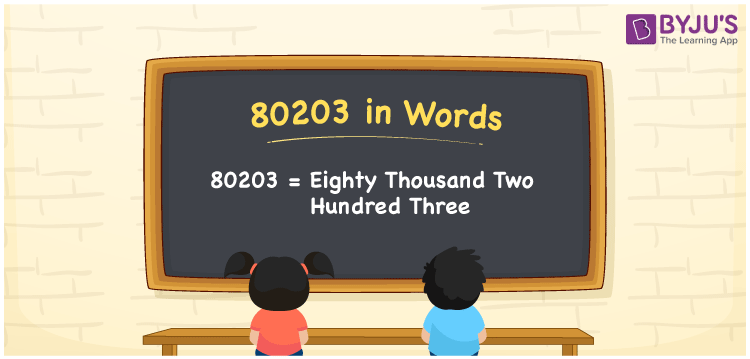# 80203 in words

80203 in words is written as Eighty Thousand Two Hundred and Three. In 80203, 8 has a place value of ten thousand, 2 is in the place value of hundred, and 3 is in the place value of one. The article onPlace Value gives more information. The number 80203 is used in expressions that relate to money, distance, length, social media views, and many more. For example, “The population of a town is Eighty Thousand Two Hundred and Three.”

 80203 in words Eighty Thousand Two Hundred and Three Eighty Thousand Two Hundred and Three in Numbers 80203

## 80203 in English Words## How to Write 80203 in Words?

We can convert 80203 to words using a place value chart. This can be done as follows. The number 80203 has 5 digits, so let’s make a chart that shows the place value up to 5 digits.

 Ten thousand Thousands Hundreds Tens Ones 8 0 2 0 3

Thus, we can write the expanded form as:

8 × Ten thousand + 0 × Thousand + 2 × Hundred + 0 × Ten + 3 × One

= 8 × 10000 + 0 × 1000 + 2 × 100 + 0 × 10 + 3 × 1

= 80203.

= Eighty Thousand Two Hundred and Three.

80203 is the natural number that is succeeded by 80202 and preceded by 80204.

80203 in words – Eighty Thousand Two Hundred and Three.

Is 80203 an odd number? – Yes.

Is 80203 an even number? – No.

Is 80203 a perfect square number? – No.

Is 80203 a perfect cube number? – No.

Is 80203 a prime number? – No.

Is 80203 a composite number? – Yes.

## Solved Example

1. Write the number 80203 in expanded form

Solution: 8 x 10000 + 0 x 1000 + 2 x 100 + 0 x 10 + 3 x 1

We can write 80203 = 80000 + 0000 + 200 + 00 + 3

= 8 x 10000 + 0 x 1000 + 2 x 100 + 0 x 10 + 3 x 1.

## Frequently Asked Questions on 80203 in words

Q1

### How to write the number 80203 in words?

80203 in words is written as Eighty Thousand Two Hundred and Three.
Q2

### Is 80203 divisible by 2?

No. 80203 is not divisible by 2.
Q3

### Is 80203 a prime number?

No. 80203 is not a prime number.